Showing:

0

312

4mos ago

8

1

MIT

# diffeqpy

diffeqpy is a package for solving differential equations in Python. It utilizes DifferentialEquations.jl for its core routines to give high performance solving of many different types of differential equations, including:

• Discrete equations (function maps, discrete stochastic (Gillespie/Markov) simulations)
• Ordinary differential equations (ODEs)
• Split and Partitioned ODEs (Symplectic integrators, IMEX Methods)
• Stochastic ordinary differential equations (SODEs or SDEs)
• Random differential equations (RODEs or RDEs)
• Differential algebraic equations (DAEs)
• Delay differential equations (DDEs)
• Mixed discrete and continuous equations (Hybrid Equations, Jump Diffusions)

directly in Python.

If you have any questions, or just want to chat about solvers/using the package, please feel free to chat in the Gitter channel. For bug reports, feature requests, etc., please submit an issue.

## Installation

To install diffeqpy, use pip:

pip install diffeqpy


Using diffeqpy requires that Julia is installed and in the path, along with DifferentialEquations.jl and PyCall.jl. To install Julia, download a generic binary from the JuliaLang site and add it to your path. To install Julia packages required for diffeqpy, open up Python interpreter then run:

>>> import diffeqpy
>>> diffeqpy.install()


and you're good! In addition, to improve the performance of your code it is recommended that you use Numba to JIT compile your derivative functions. To install Numba, use:

pip install numba


## General Flow

Import and setup the solvers available in DifferentialEquations.jl via the commands:

from diffeqpy import de


In case only the solvers available in OrdinaryDiffEq.jl are required then use the command:

from diffeqpy import ode


The general flow for using the package is to follow exactly as would be done in Julia, except add de. or ode. in front. Note that ode. has lesser loading time and a smaller memory footprint compared to de.. Most of the commands will work without any modification. Thus the DifferentialEquations.jl documentation and the DiffEqTutorials are the main in-depth documentation for this package. Below we will show how to translate these docs to Python code.

Python does not allow ! in function names, so this is also a limitation of pyjulia To use functions which on the Julia side have a !, like step!, replace ! by _b, for example:

from diffeqpy import de
def f(u,p,t):
return -u
u0 = 0.5
tspan = (0., 1.)
prob = de.ODEProblem(f, u0, tspan)
integrator = de.init(prob, de.Tsit5())
de.step_b(integrator)


is valid Python code for using the integrator interface.

## Ordinary Differential Equation (ODE) Examples

### One-dimensional ODEs

from diffeqpy import de

def f(u,p,t):
return -u

u0 = 0.5
tspan = (0., 1.)
prob = de.ODEProblem(f, u0, tspan)
sol = de.solve(prob)


The solution object is the same as the one described in the DiffEq tutorials and in the solution handling documentation (note: the array interface is missing). Thus for example the solution time points are saved in sol.t and the solution values are saved in sol.u. Additionally, the interpolation sol(t) gives a continuous solution.

We can plot the solution values using matplotlib:

import matplotlib.pyplot as plt
plt.plot(sol.t,sol.u)
plt.show()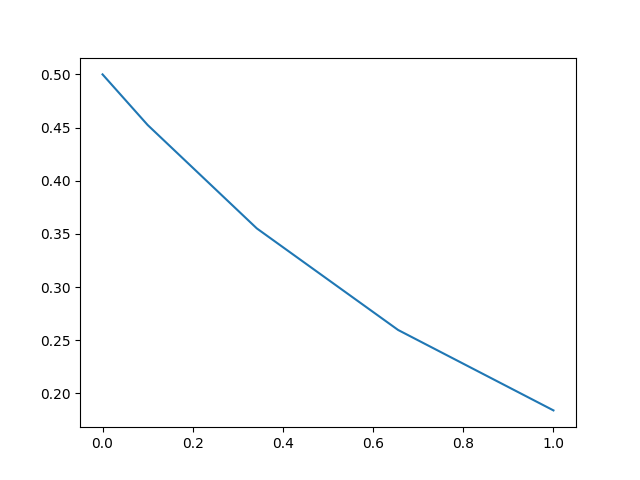We can utilize the interpolation to get a finer solution:

import numpy
t = numpy.linspace(0,1,100)
u = sol(t)
plt.plot(t,u)
plt.show()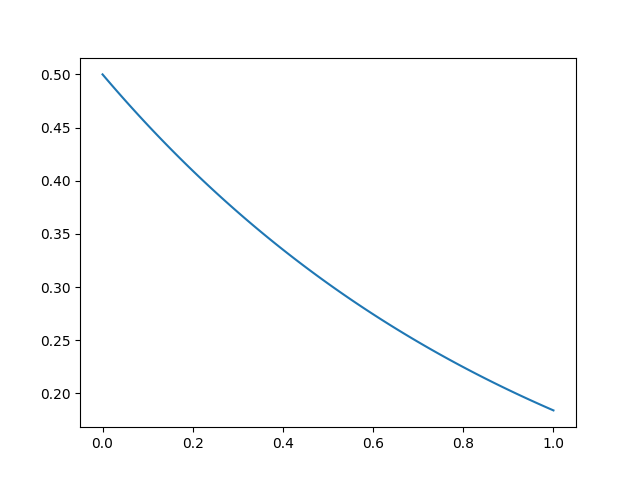### Solve commands

The common interface arguments can be used to control the solve command. For example, let's use saveat to save the solution at every t=0.1, and let's utilize the Vern9() 9th order Runge-Kutta method along with low tolerances abstol=reltol=1e-10:

sol = de.solve(prob,de.Vern9(),saveat=0.1,abstol=1e-10,reltol=1e-10)


The set of algorithms for ODEs is described at the ODE solvers page.

### Compilation with Numba and Julia

When solving a differential equation, it's pertinent that your derivative function f is fast since it occurs in the inner loop of the solver. We can utilize Numba to JIT compile our derivative functions to improve the efficiency of the solver:

import numba
numba_f = numba.jit(f)

prob = de.ODEProblem(numba_f, u0, tspan)
sol = de.solve(prob)


Additionally, you can directly define the functions in Julia. This will allow for more specialization and could be helpful to increase the efficiency over the Numba version for repeat or long calls. This is done via julia.Main.eval:

from julia import Main
jul_f = Main.eval("(u,p,t)->-u") # Define the anonymous function in Julia
prob = de.ODEProblem(jul_f, u0, tspan)
sol = de.solve(prob)


### Systems of ODEs: Lorenz Equations

To solve systems of ODEs, simply use an array as your initial condition and define f as an array function:

def f(u,p,t):
x, y, z = u
sigma, rho, beta = p
return [sigma * (y - x), x * (rho - z) - y, x * y - beta * z]

u0 = [1.0,0.0,0.0]
tspan = (0., 100.)
p = [10.0,28.0,8/3]
prob = de.ODEProblem(f, u0, tspan, p)
sol = de.solve(prob,saveat=0.01)

plt.plot(sol.t,sol.u)
plt.show()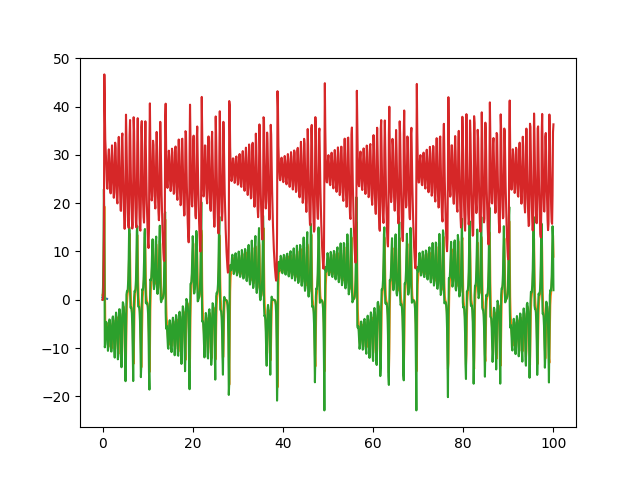or we can draw the phase plot:

ut = numpy.transpose(sol.u)
from mpl_toolkits.mplot3d import Axes3D
fig = plt.figure()
ax.plot(ut[0,:],ut[1,:],ut[2,:])
plt.show()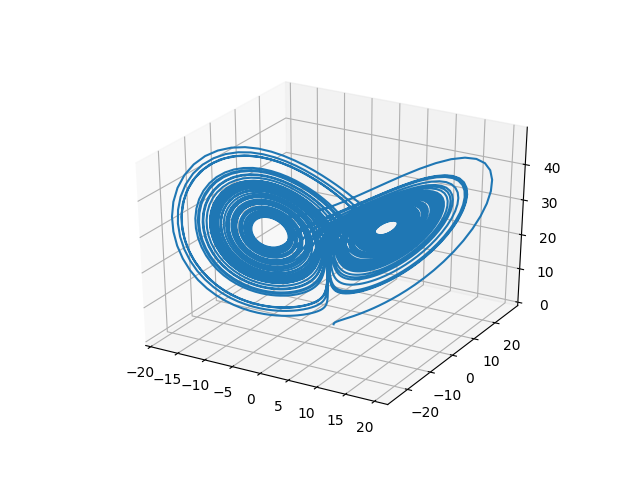### In-Place Mutating Form

When dealing with systems of equations, in many cases it's helpful to reduce memory allocations by using mutating functions. In diffeqpy, the mutating form adds the mutating vector to the front. Let's make a fast version of the Lorenz derivative, i.e. mutating and JIT compiled:

def f(du,u,p,t):
x, y, z = u
sigma, rho, beta = p
du = sigma * (y - x)
du = x * (rho - z) - y
du = x * y - beta * z

numba_f = numba.jit(f)
u0 = [1.0,0.0,0.0]
tspan = (0., 100.)
p = [10.0,28.0,2.66]
prob = de.ODEProblem(numba_f, u0, tspan, p)
sol = de.solve(prob)


or using a Julia function:

jul_f = Main.eval("""
function f(du,u,p,t)
x, y, z = u
sigma, rho, beta = p
du = sigma * (y - x)
du = x * (rho - z) - y
du = x * y - beta * z
end""")
u0 = [1.0,0.0,0.0]
tspan = (0., 100.)
p = [10.0,28.0,2.66]
prob = de.ODEProblem(jul_f, u0, tspan, p)
sol = de.solve(prob)


## Stochastic Differential Equation (SDE) Examples

### One-dimensional SDEs

Solving one-dimensonal SDEs du = f(u,t)dt + g(u,t)dW_t is like an ODE except with an extra function for the diffusion (randomness or noise) term. The steps follow the SDE tutorial.

def f(u,p,t):
return 1.01*u

def g(u,p,t):
return 0.87*u

u0 = 0.5
tspan = (0.0,1.0)
prob = de.SDEProblem(f,g,u0,tspan)
sol = de.solve(prob,reltol=1e-3,abstol=1e-3)

plt.plot(sol.t,sol.u)
plt.show()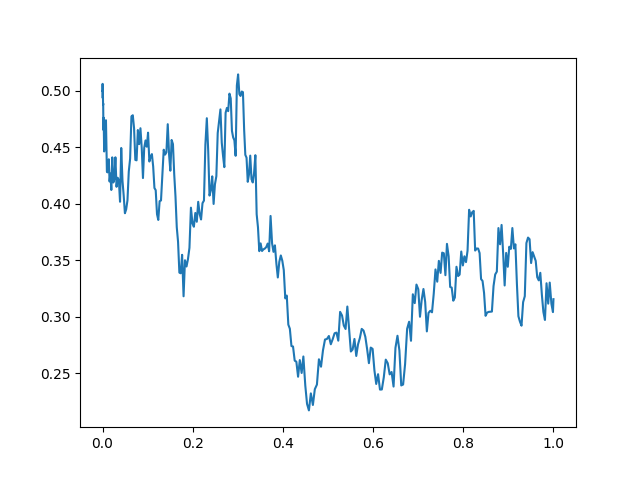### Systems of SDEs with Diagonal Noise

An SDE with diagonal noise is where a different Wiener process is applied to every part of the system. This is common for models with phenomenological noise. Let's add multiplicative noise to the Lorenz equation:

def f(du,u,p,t):
x, y, z = u
sigma, rho, beta = p
du = sigma * (y - x)
du = x * (rho - z) - y
du = x * y - beta * z

def g(du,u,p,t):
du = 0.3*u
du = 0.3*u
du = 0.3*u

numba_f = numba.jit(f)
numba_g = numba.jit(g)
u0 = [1.0,0.0,0.0]
tspan = (0., 100.)
p = [10.0,28.0,2.66]
prob = de.SDEProblem(numba_f, numba_g, u0, tspan, p)
sol = de.solve(prob)

# Now let's draw a phase plot

ut = numpy.transpose(sol.u)
from mpl_toolkits.mplot3d import Axes3D
fig = plt.figure()
ax.plot(ut[0,:],ut[1,:],ut[2,:])
plt.show()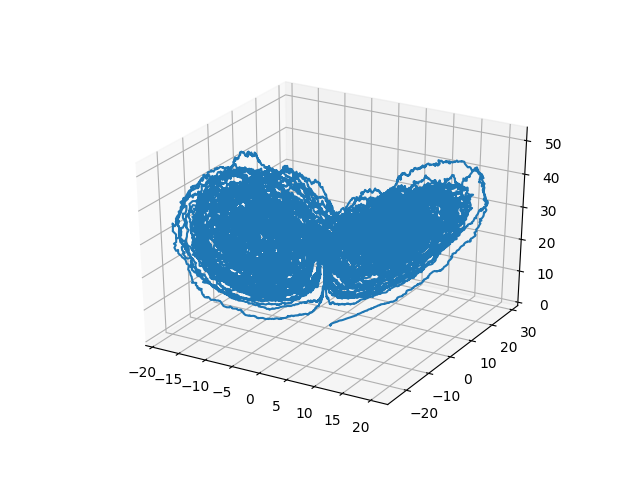### Systems of SDEs with Non-Diagonal Noise

In many cases you may want to share noise terms across the system. This is known as non-diagonal noise. The DifferentialEquations.jl SDE Tutorial explains how the matrix form of the diffusion term corresponds to the summation style of multiple Wiener processes. Essentially, the row corresponds to which system the term is applied to, and the column is which noise term. So du[i,j] is the amount of noise due to the jth Wiener process that's applied to u[i]. We solve the Lorenz system with correlated noise as follows:

def f(du,u,p,t):
x, y, z = u
sigma, rho, beta = p
du = sigma * (y - x)
du = x * (rho - z) - y
du = x * y - beta * z

def g(du,u,p,t):
du[0,0] = 0.3*u
du[1,0] = 0.6*u
du[2,0] = 0.2*u
du[0,1] = 1.2*u
du[1,1] = 0.2*u
du[2,1] = 0.3*u

u0 = [1.0,0.0,0.0]
tspan = (0.0,100.0)
p = [10.0,28.0,2.66]
nrp = numpy.zeros((3,2))
numba_f = numba.jit(f)
numba_g = numba.jit(g)
prob = de.SDEProblem(numba_f,numba_g,u0,tspan,p,noise_rate_prototype=nrp)
sol = de.solve(prob,saveat=0.005)

# Now let's draw a phase plot

ut = numpy.transpose(sol.u)
from mpl_toolkits.mplot3d import Axes3D
fig = plt.figure()
ax.plot(ut[0,:],ut[1,:],ut[2,:])
plt.show()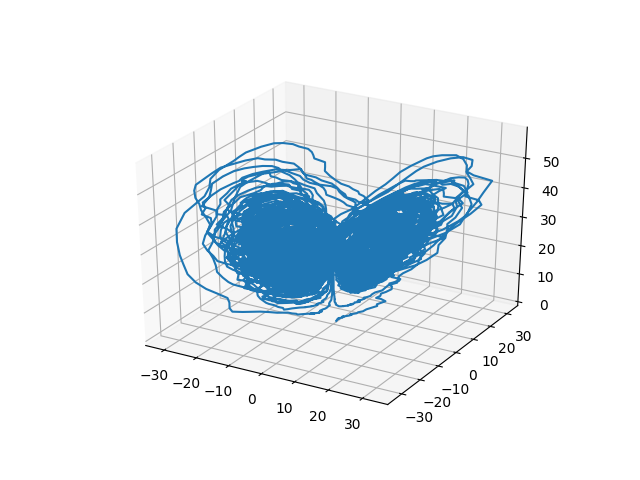Here you can see that the warping effect of the noise correlations is quite visible!

## Differential-Algebraic Equation (DAE) Examples

A differential-algebraic equation is defined by an implicit function f(du,u,p,t)=0. All of the controls are the same as the other examples, except here you define a function which returns the residuals for each part of the equation to define the DAE. The initial value u0 and the initial derivative du0 are required, though they do not necessarily have to satisfy f (known as inconsistent initial conditions). The methods will automatically find consistent initial conditions. In order for this to occur, differential_vars must be set. This vector states which of the variables are differential (have a derivative term), with false meaning that the variable is purely algebraic.

This example shows how to solve the Robertson equation:

def f(du,u,p,t):
resid1 = - 0.04*u               + 1e4*u*u - du
resid2 = + 0.04*u - 3e7*u**2 - 1e4*u*u - du
resid3 = u + u + u - 1.0
return [resid1,resid2,resid3]

u0 = [1.0, 0.0, 0.0]
du0 = [-0.04, 0.04, 0.0]
tspan = (0.0,100000.0)
differential_vars = [True,True,False]
prob = de.DAEProblem(f,du0,u0,tspan,differential_vars=differential_vars)
sol = de.solve(prob)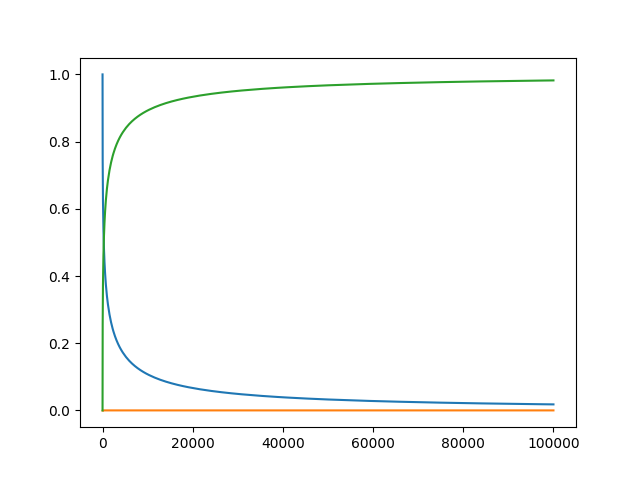and the in-place JIT compiled form:

def f(resid,du,u,p,t):
resid = - 0.04*u               + 1e4*u*u - du
resid = + 0.04*u - 3e7*u**2 - 1e4*u*u - du
resid = u + u + u - 1.0

numba_f = numba.jit(f)
prob = de.DAEProblem(numba_f,du0,u0,tspan,differential_vars=differential_vars)
sol = de.solve(prob)


## Delay Differential Equations

A delay differential equation is an ODE which allows the use of previous values. In this case, the function needs to be a JIT compiled Julia function. It looks just like the ODE, except in this case there is a function h(p,t) which allows you to interpolate and grab previous values.

We must provide a history function h(p,t) that gives values for u before t0. Here we assume that the solution was constant before the initial time point. Additionally, we pass constant_lags = [20.0] to tell the solver that only constant-time lags were used and what the lag length was. This helps improve the solver accuracy by accurately stepping at the points of discontinuity. Together this is:

f = Main.eval("""
function f(du, u, h, p, t)
du = 1.1/(1 + sqrt(10)*(h(p, t-20))^(5/4)) - 10*u/(1 + 40*u)
du = 100*u/(1 + 40*u) - 2.43*u
end""")
u0 = [1.05767027/3, 1.030713491/3]

h = Main.eval("""
function h(p,t)
[1.05767027/3, 1.030713491/3]
end
""")

tspan = (0.0, 100.0)
constant_lags = [20.0]
prob = de.DDEProblem(f,u0,h,tspan,constant_lags=constant_lags)
sol = de.solve(prob,saveat=0.1)

u1 = [sol.u[i] for i in range(0,len(sol.u))]
u2 = [sol.u[i] for i in range(0,len(sol.u))]

import matplotlib.pyplot as plt
plt.plot(sol.t,u1)
plt.plot(sol.t,u2)
plt.show()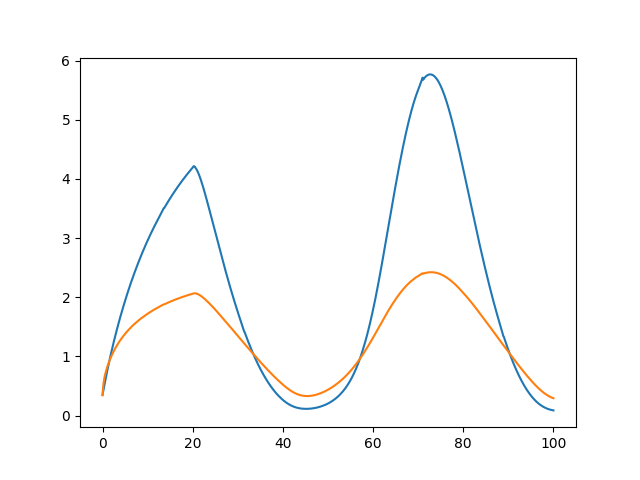Notice that the solver accurately is able to simulate the kink (discontinuity) at t=20 due to the discontinuity of the derivative at the initial time point! This is why declaring discontinuities can enhance the solver accuracy.

## Known Limitations

• Autodiff does not work on Python functions. When applicable, either define the derivative function as a Julia function or set the algorithm to use finite differencing, i.e. Rodas5(autodiff=false). All default methods use autodiff.
• Delay differential equations have to use Julia-defined functions otherwise the history function is not appropriately typed with the overloads.

## Testing

Unit tests can be run by tox.

tox
tox -e py3-numba   # test with Numba


### Troubleshooting

In case you encounter silent failure from tox, try running it with -- -s (e.g., tox -e py36 -- -s) where -s option (--capture=no, i.e., don't capture stdio) is passed to py.test. It may show an error message "error initializing LibGit2 module". In this case, setting environment variable SSL_CERT_FILE may help; e.g., try:

SSL_CERT_FILE=PATH/TO/cert.pem tox -e py36


## Rate & Review

Great Documentation0
Easy to Use0
Performant0
Highly Customizable0
Bleeding Edge0
Responsive Maintainers0
Poor Documentation0
Hard to Use0
Slow0
Buggy0
Abandoned0
Unwelcoming Community0
100